# ISEE Middle Level Math : Distributive Property

## Example Questions

### Example Question #656 : Numbers And Operations

Which equation demonstrates the distributive property?

Possible Answers: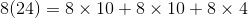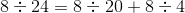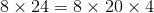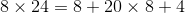Correct answer:Explanation:

The distributive property is explained in the following equation: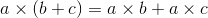It is important to remember that the distributive property does not apply to division.

### Example Question #657 : Numbers And Operations

Simplify the following algebraic expression: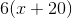Possible Answers: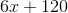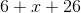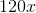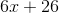Correct answer:Explanation:

According to the distributive property, when you multiply a number or variable by the sum of a group of numbers or variables, you will get the same answer as multiplying each number or variable separately and then adding the products together.

It is represented by the following equation:Therefore, in this question: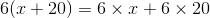or### Example Question #658 : Numbers And Operations

Solve using the distributive property: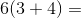Possible Answers:Correct answer:Explanation:

First multiply 6 by each number in parentheses: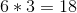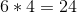Then solve equation: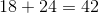Answer:### Example Question #659 : Numbers And Operations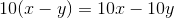, is this expression true under the distributive property?

Possible Answers: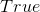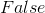Correct answer:Explanation:

The distributive property says that when a value is multiplied by a parentheses, you multiply the value be each value in the parentheses.

For this expression you dotimesand also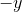giving you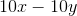.

### Example Question #660 : Numbers And Operations

What is an equivalent expression to the following expression?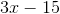Possible Answers: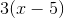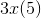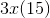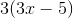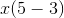Correct answer:Explanation:

For this expression, you can undue the distributive property and take out a common term instead.

They have a common factor ofso you can divide both by that value and put it outside a set of parantheses around the expression.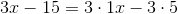Your final answer would look like this:.

### Example Question #91 : Distributive Property

Find the mean in this set of numbers: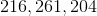Possible Answers: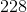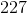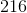Correct answer:Explanation:

To solve , first add all the numbers in the set: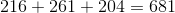Then divide by the amount of numbers in the set: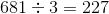Answer: The mean is### Example Question #92 : Distributive Property

Solve using the distributive property: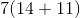Possible Answers: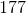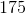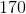Correct answer:Explanation:

To solve, first multiply each number in parenthesis by 7: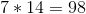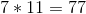Then, solve the equation: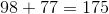Answer:### Example Question #93 : Distributive Property

Solve using the distributive property: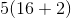Possible Answers:Correct answer:Explanation:

To solve: first multiply the number outside the brackets.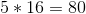Then multiply: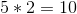Then add the products: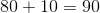### Example Question #94 : Distributive Property

Solve using the distributive property: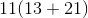Possible Answers: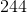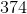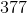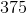Correct answer:Explanation:

To solve, first multiply: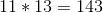Then, multiply: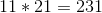Finally, add the products: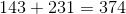### Example Question #95 : Distributive Property

Solve using the distributive property: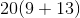Possible Answers: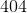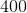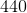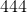Correct answer:Explanation:

To solve, multiply each number in parenthesis by 20: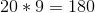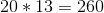Then, solve the equation: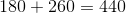### All ISEE Middle Level Math Resources